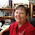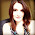## Thursday, May 2, 2013

### Adventures in Monotangles #9 – ‘NZEPPEL

Well this is week nine, where does the tie go. This week has been interesting. Over the weekend I spent way too much time on Tanglepattens.com, deciding which tangle to use this week. As number nine is one of my favorite numbers I wanted something special. Well yesterday I sat down, to do a tile for the adventure, and started tangling.  As can happen in Zentangle® what came out of my pen was not the Tangle I planned. So I will postpone the previously chosen tangle, and allow ‘Nzepple to be the pattern for this week. I really love Nzepple as a tangle, so I am in no way surprised this happened. I ended up doing a large tangle, then filled each section of the tangle with a smaller version of the tangle, I really like how this turned out.

If you have not done ‘Nzepple before, you can find out more about it Here.

And here is My ‘Nzeppel Zentangle® Tile!

Some of the reasons I like the number nine… (Yoinked from Wikipedia)

Nine is a composite number, its proper divisors being 1 and 3. It is 3 times 3 and hence the third square number. Nine is a Motzkin number. It is the first composite lucky number, along with the first composite odd number.

Nine is the highest single-digit number in the decimal system. It is the second non-unitary square prime of the form (p2) and the first that is odd. All subsequent squares of this form are odd. It has a unique aliquot sum 4 which is itself a square prime. Nine is; and can be, the only square prime with an aliquot sum of the same form. The aliquot sequence of nine has 5 members (9,4,3,1,0) this number being the second composite member of the 3-aliquot tree. It is the aliquot sum of only one number the discrete semiprime 15.
There are nine Heegner numbers.

Since 9 = 321, 9 is an exponential factorial.

8 and 9 form a Ruth-Aaron pair under the second definition that counts repeated prime factors as often as they occur.

In bases 12, 18 and 24, nine is a 1-automorphic number and in base 6 a 2-automorphic number (displayed as '13').

A polygon with nine sides is called a nonagon or enneagon] A group of nine of anything is called an ennead.
In base 10 a number is divisible by nine if and only if its digital root is 9. That is, if you multiply nine by any natural number, and repeatedly add the digits of the answer until it is just one digit, you will end up with nine:
2 × 9 = 18 (1 + 8 = 9)
3 × 9 = 27 (2 + 7 = 9)
9 × 9 = 81 (8 + 1 = 9)
121 × 9 = 1089 (1 + 0 + 8 + 9 = 18; 1 + 8 = 9)
234 × 9 = 2106 (2 + 1 + 0 + 6 = 9)
578329 × 9 = 5204961 (5 + 2 + 0 + 4 + 9 + 6 + 1 = 27; 2 + 7 = 9)
482729235601 × 9 = 4344563120409 (4 + 3 + 4 + 4 + 5 + 6 + 3 + 1 + 2 + 0 + 4 + 0 + 9 = 45; 4 + 5 = 9)

There are other interesting patterns involving multiples of nine:
12345679 x 9 = 111111111
12345679 x 18 = 222222222
12345679 x 81 = 999999999

This works for all the multiples of 9. n = 3 is the only other n > 1 such that a number is divisible by n if and only if its digital root is n. In base N, the divisors of N − 1 have this property. Another consequence of 9 being 10 − 1, is that it is also a Kaprekar number.
The difference between a base-10 positive integer and the sum of its digits is a whole multiple of nine. Examples:
The sum of the digits of 41 is 5, and 41-5 = 36. The digital root of 36 is 3+6 = 9, which, as explained above, demonstrates that it is divisible by nine.
The sum of the digits of 35967930 is 3+5+9+6+7+9+3+0 = 42, and 35967930-42 = 35967888. The digital root of 35967888 is 3+5+9+6+7+8+8+8 = 54, 5+4 = 9.
Subtracting two base-10 positive integers that are transpositions of each other yields a number that is a whole multiple of nine. Examples:
41 - 14 = 27 (2 + 7 = 9)

36957930 - 35967930 = 990000, a multiple of nine.
This works regardless of the number of digits that are transposed. For example, the largest transposition of 35967930 is 99765330 (all digits in descending order) and its smallest transposition is 03356799 (all digits in ascending order); subtracting pairs of these numbers produces:
99765330 - 35967930 = 63797400; 6+3+7+9+7+4+0+0 = 36; 3+6 = 9.
99765330 - 03356799 = 96408531; 9+6+4+0+8+5+3+1 = 36; 3+6 = 9.
35967930 - 03356799 = 32611131; 3+2+6+1+1+1+3+1 = 18; 1+8 = 9.

Casting out nines is a quick way of testing the calculations of sums, differences, products, and quotients of integers, known as long ago as the 12th Century.

Every prime in a Cunningham chain of the first kind with a length of 4 or greater is congruent to 9 mod 10 (the only exception being the chain 2, 5, 11, 23, 47).

Six recurring nines appear in the decimal places 762 through 767 of pi. This is known as the Feynman point.
If an odd perfect number is of the form 36k + 9, it has at least nine distinct prime factors.
If you divide a number by the amount of 9s corresponding to its number of digits, the number is turned into a repeating decimal. (e.g. 274/999 = 0.274274274274...)

Nine is the binary complement of number six:
9 = 1001
6 = 0110

1.Like your shading and the stippling that you did. 'Nzeppel is one of my favorite tangles.

1.Sorry about the double link. Hit the wrong key. The one for this challenge is #4

2.I love your 'Nzeppel Roy. I like the (vague) contrast.
And I owe you a BIG thank you for making me draw this difficult tangle.

3.I am glad that you kind of, nicely of course, force us into doing somethings that we don't particularly like. I think we don't like them because we can't do them. This challenge makes us pay attention, and then we find out we actually like the tangle. Thanks again also, Roy, for being so kind about my sensitive ears :)

4.I don't think this will ever be one of my favorite tangles, but I'm like Jean, I have to thank you Roy for making me think outside my comfort zone and learn something new to me. That's one reason I like to participate in challenges they keep me from falling into only what comfortable.

5.Thanks again, Roy! I really enjoy your blog :)

6.All done! This one was more challenging to get a unique take.

7.One of my favourite tangles - thanks Roy, had fun with this one :)

8.Thanks for introducing to me to this tangle! It has quickly become a new favorite of mine.

9.and I didn't mean to double link. I thought I mis-clicked the first time. If you're able to delete entries go ahead and delete my first one. It was the wrong link anyway. It jumps to my comment section instead of the top of my post.